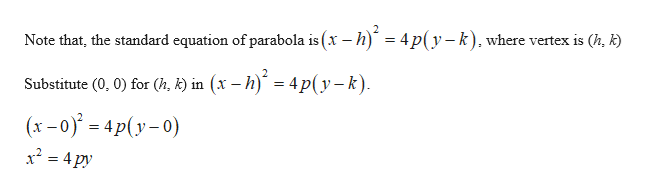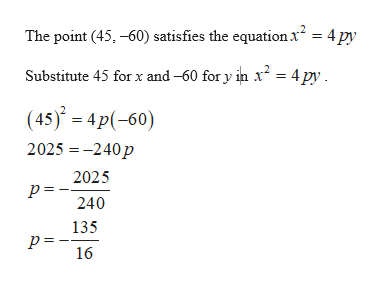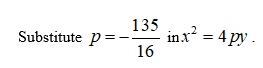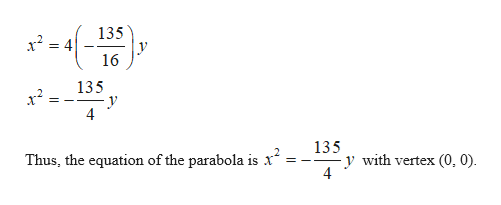# Two large airship hangars were built in Orly, France, in the early 1900s but were destroyed in World War II by American aircraft. The hangars were 175 m in length, 90 m wide, and 60 m high and were formed by a series of parabolic arches.a. Set up a coordinate system with (0, 0) at the vertex of one of the arches and write an equation of the parabola.b. What is the focal length of an arch?

Question
159 views

Two large airship hangars were built in Orly, France, in the early 1900s but were destroyed in World War II by American aircraft. The hangars were 175 m in length, 90 m wide, and 60 m high and were formed by a series of parabolic arches.

a. Set up a coordinate system with (0, 0) at the vertex of one of the arches and write an equation of the parabola.

b. What is the focal length of an arch?

check_circle

Step 1

(a). It is given that, the length of hangars is 175 m, the width of the hangers is 90 m and the height of the hangers is 60 m.

The vertex of the parabola is (0, 0).help_outlineImage TranscriptioncloseNote that, the standard equation of parabola is (x – h) = 4p(y–k), where vertex is (h, k) Substitute (0, 0) for (h, k) in (x – h) = 4p(y–k). (x –0) = 4p(y- 0) x² = 4 py fullscreen
Step 2

Since the width of the parabola is 90, which means the points that lies on the parabola.help_outlineImage TranscriptioncloseThe point (45, -60) satisfies the equation x = 4 py Substitute 45 for x and -60 for y in x² = 4 py. (45) = 4p(-60) 2025 = -240p 2025 p = -. 240 135 16 fullscreen
Step 3...help_outlineImage Transcriptionclose135 x² = 16 135 x? 135 y with vertex (0, 0). 4 Thus, the equation of the parabola is x fullscreen

### Want to see the full answer?

See Solution

#### Want to see this answer and more?

Solutions are written by subject experts who are available 24/7. Questions are typically answered within 1 hour.*

See Solution
*Response times may vary by subject and question.
Tagged in

### Other